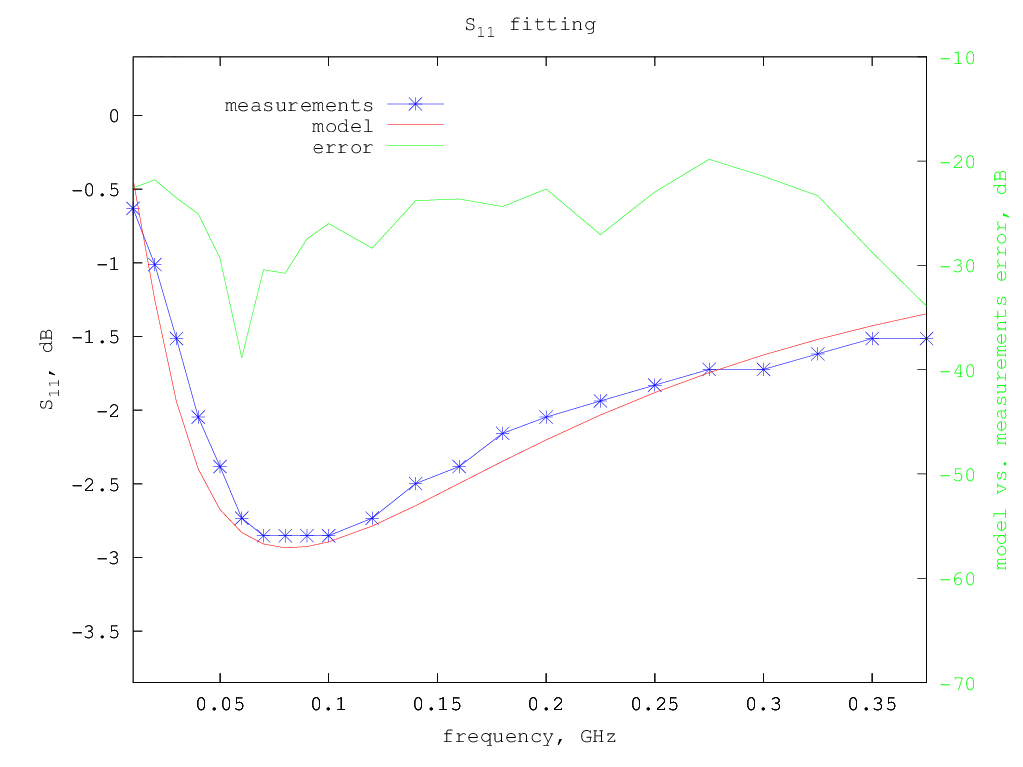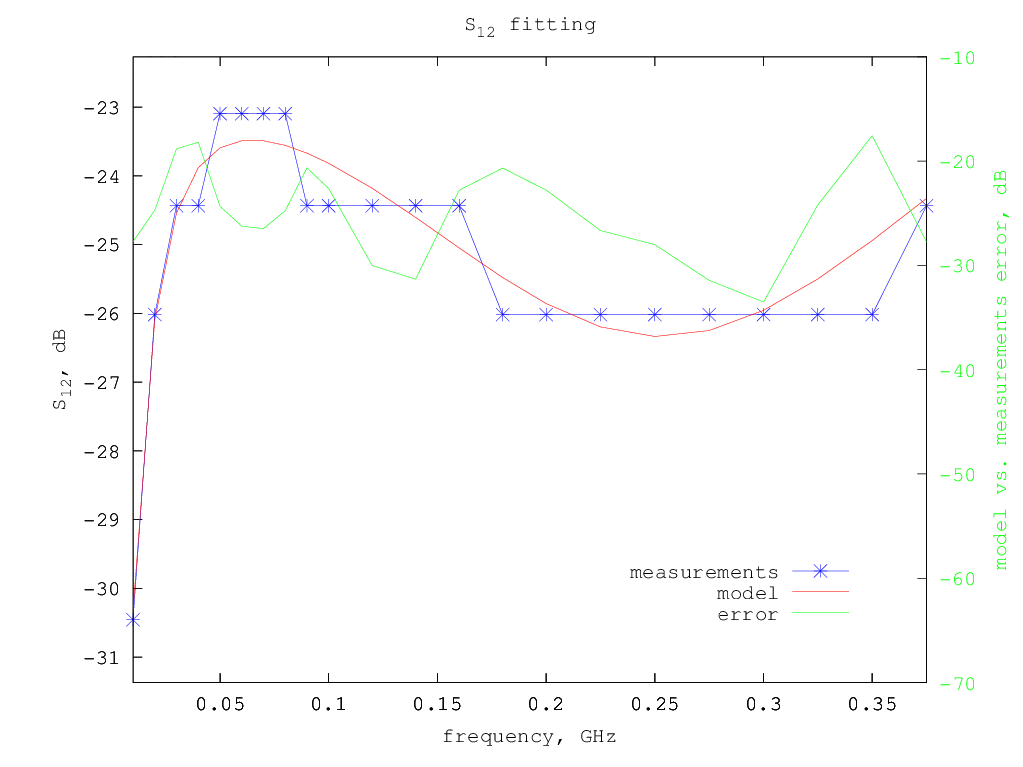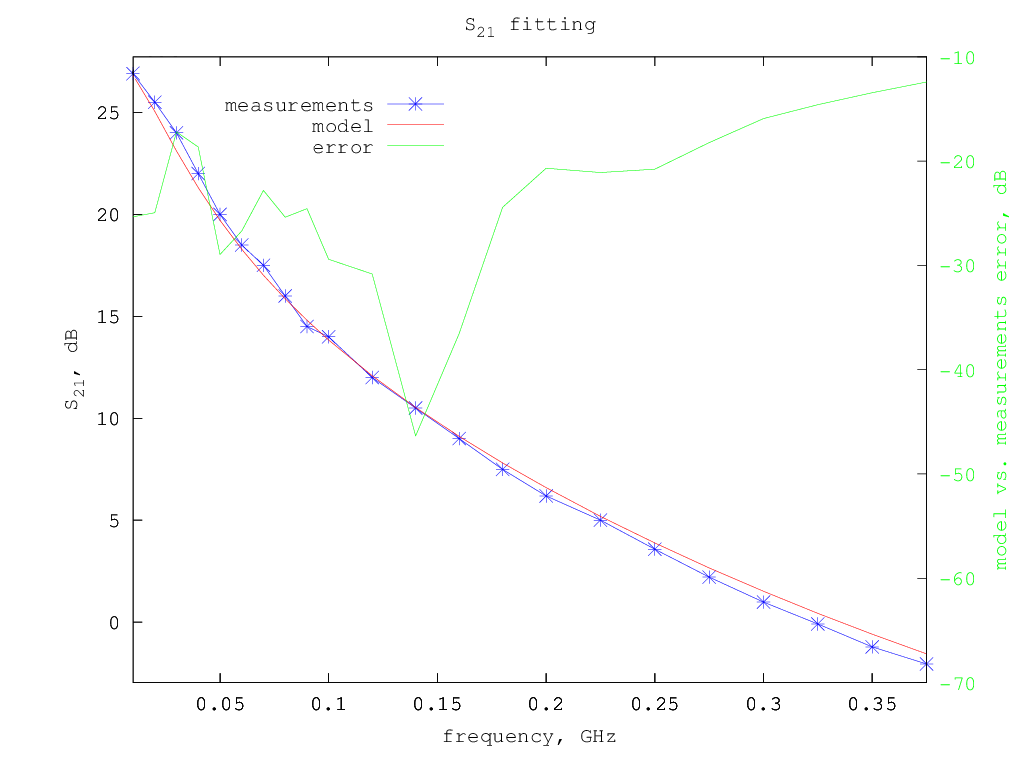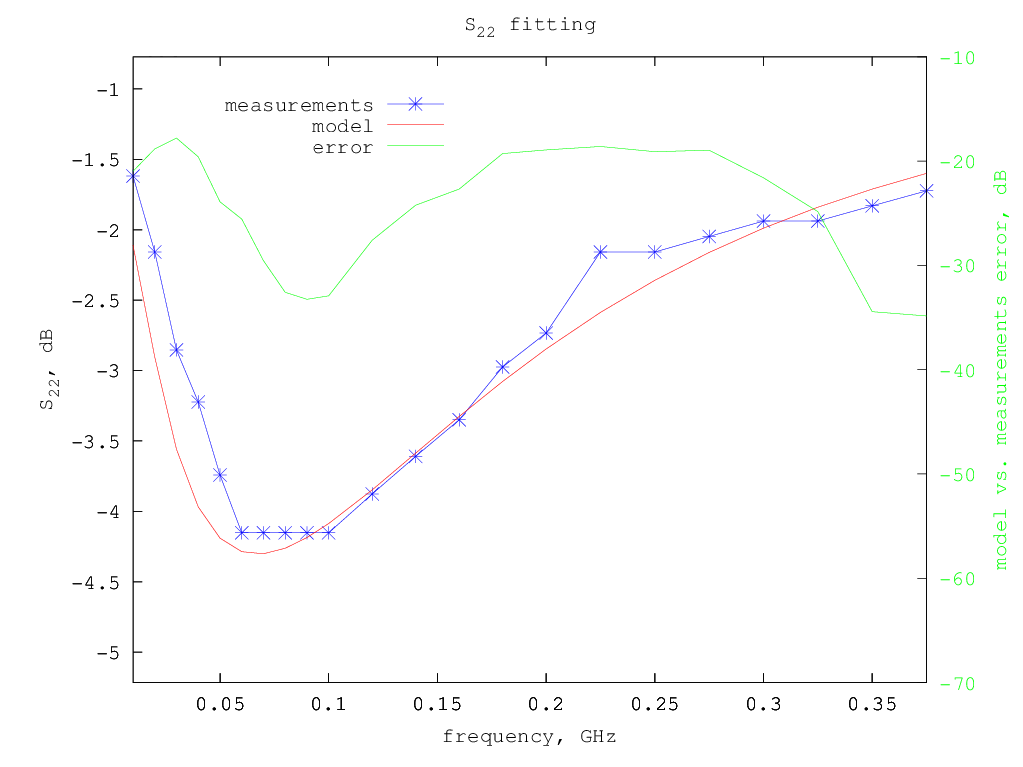# Siliconix VMP4 LDMOS model

Under construction...

The VMP4 was a power LDMOS manufactured by Siliconix a lot of time ago; it was used by amateur radio experimenters as a medium power amplifier or sometimes even as high-performance preamplifier for radio receivers.
The modeling done here might be interesting to further analyze some old circuits and to compare this ancient LDMOS with more modern devices, like the RD16HHF1.

# Model for Vdd=28 V and Idd= 450 mA

Typical S-parameters for this device are in the datasheet that can still be found on the 'net, for a supply voltage of 28 V and a drain current of 450 mA. Unfortunately the data are given only in a short table form, so not many points are available.

Using the extraction method previously described, and considering the full available S-parameters range (10 MHz-375 MHz) the following small-signal model parameters are determined:

```Rg = 1.05 ohm
Rd = 0.995 ohm
Rs = 0.917 ohm
Lg = 4.216 nH
Ld = 2.552 nH
Ls = 0.924 nH
Cgs = 51.7 pF
Cds = 39.1 pF
Cgd = 5.75 pF
gm = 0.35 S
gds = 2.57 mS
```
So the equivalent small-signal circuit for this LDMOS at 450 mA drain current is the following:Smith chart with measured vs. model S-parameters: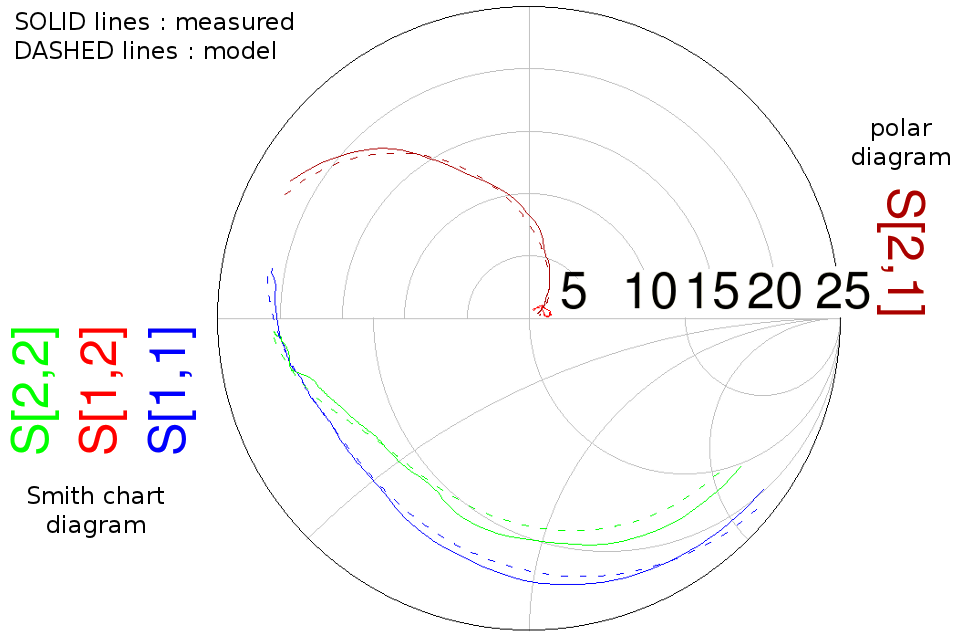S-parameters fitting details - whole frequency span was considered for fitting: